Try Magic Notes and save time.Try it free
Try Magic Notes and save timeCrush your year with the magic of personalized studying.Try it free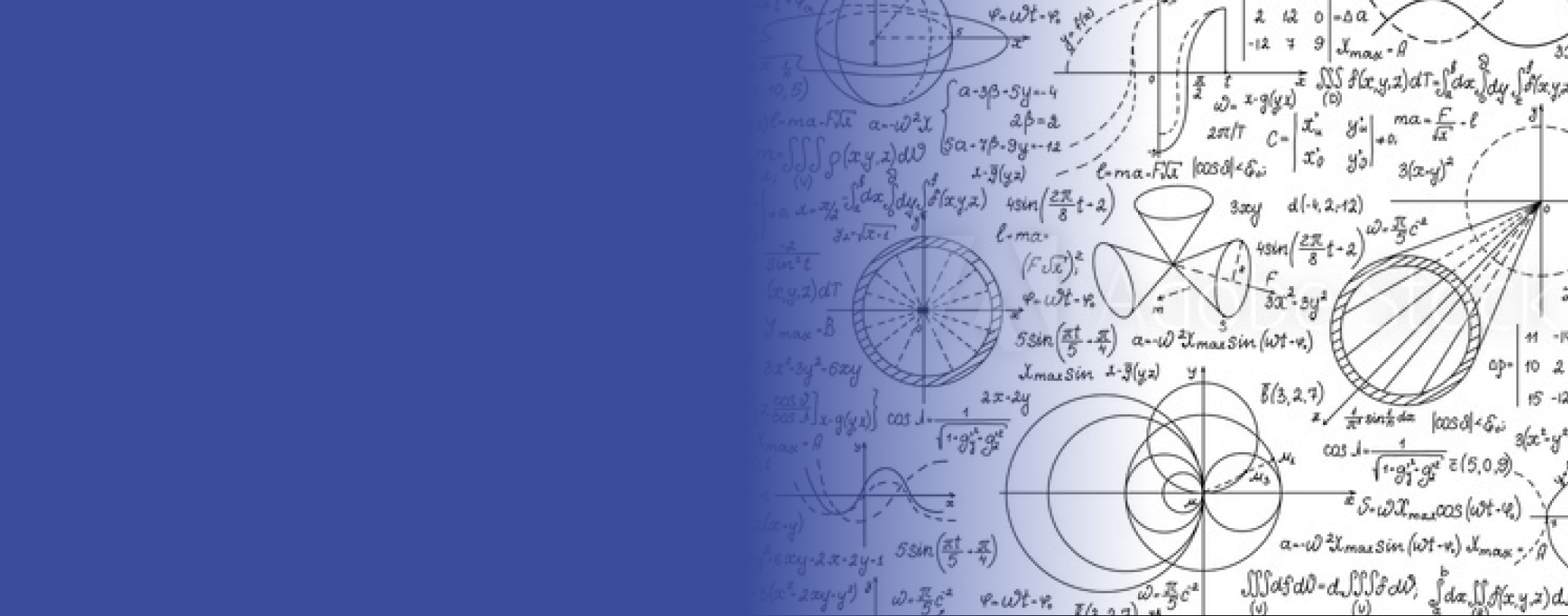# Geometry Lesson Plans: 7 Discovery & Smart Learning Ideas

Want to make a math topic stick? Whether you’re talking about algebra, geometry or trigonometry, don’t just teach your students the correct material. Providing opportunities to learn that material in the right way will maximize their chances of fully comprehending and remembering it.

Experiencing a concept by doing helps a student master the information. In addition to simply reading the information, the student creates a memory of a hands-on activity that will supplement the information acquired from a textbook. This boosts the student’s chances of retaining that information and being able to recall it and put it to use when needed.

Geometry is a subject that is very well-suited to this sort of learning. Here are seven, simple, but effective, hands-on activities to use in your geometry class or unit. These activities work well in a classroom setting and can be adapted for distance learning.

## 1. Discover the Triangle Angle Sum Theorem

One of the first theorems that most geometry students learn is the Triangle Angle Sum Theorem.  According to this theorem, the sum of the angles in a triangle is 180°.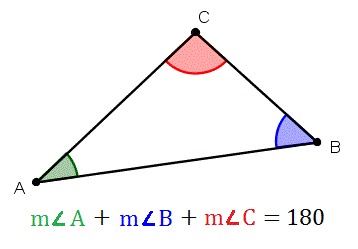Image Source

You could simply tell your class that this theorem is true. But if you want to help your students master the material, allow them to discover it on their own.

Here’s how:

Step 1: Have each student cut out a triangle. The students may draw their own triangles, or the teacher may provide pre-drawn triangles of different shapes and sizes.

Step 2: Ask the students to mark each corner of their triangles with a letter, number, dot or other mark.

Step 3: Have the students cut off the corners of their triangles.

Step 4: Finally, ask the students to abut the three corners together. This will result in a straight line no matter how the angles are arranged.  A straight line equals 180°, so this activity demonstrates that the sum of a triangle’s angles is always 180°.

Tips for success: Encourage students to rearrange their corners in different ways to see that any arrangement of the pieces will create a straight line. Ask them to look at their classmates’ work, as well. Since each student starts with a different triangle, the fact that this activity works in every instance will reinforce that the Triangle Angle Sum Theorem is true for every triangle.

In distance learning situations, students may upload photos of their work or glue their pieces to a sheet that they can hold up for their classmates to see during a virtual class session.

## 2. Discover the Quadrilateral Sum Conjecture

The Quadrilateral Sum Conjecture states that the sum of the angles of a convex quadrilateral is 360°. We can demonstrate this conjecture .  much the same way as we did the previous theorem.  Here’s how to do it:

Step 1: Have each student cut out a convex quadrilateral of any kind. Again, the students may draw their own quadrilaterals or the teacher may hand out pre-drawn quadrilaterals of different shapes and sizes.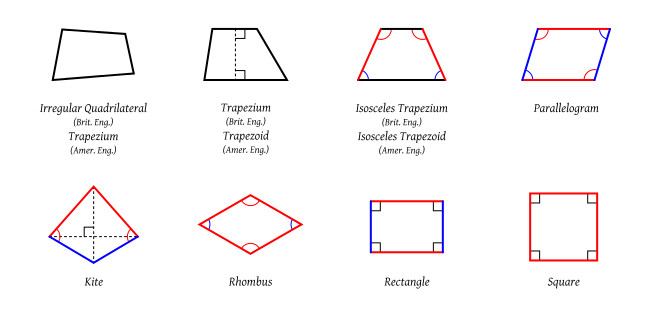Image Source

Step 2: Ask the students to mark each corner of their quadrilaterals with a letter, number, dot or other mark.

Step 3: Have the students cut off the corners of their quadrilaterals.

Step 4: Ask the students to take the four corners and arrange them with the marks together. No matter how they are arranged, the pieces will meet to form a circle.

Because a circle equals 360°, this activity demonstrates that the sum of the four angles of a quadrilateral is always 360°.

Tips for success: Again, have the students rearrange their corners, marks together, to show that any arrangement creates a circle at a point. When the students examine their classmates’ work, they should see that this works for all of the unique quadrilaterals in the class. As with the Triangle Angle Sum activity, teachers may adapt this for distance learning by asking students to show their classmates their work during a virtual session, or upload their work for their classmates to review.

## 3. Demonstrate That the Area Formulas for Rectangles and Parallelograms Are the Same

Geometry students learn that the areas of both rectangles and parallelograms  are calculated as follows:

Area=base × height, or A=bh

Most students have little trouble understanding why the formula for a rectangle works. For example, if we have a rectangle with a base of 4 cm and a height of 3 cm , we can draw grid lines that show the area to be 12 cm2, like this: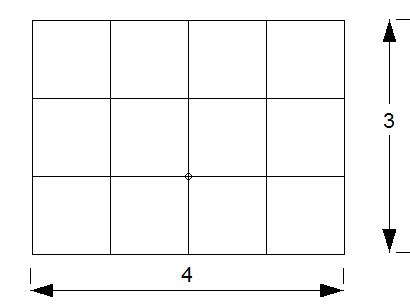But, it can be trickier to show why this formula can be used to calculate area for a parallelogram.

So, what to do? Turn a parallelogram into a rectangle! Here’s how to do it:

[Imbed video: https://virtualnerd.com/geometry/length-area/parallelogram-triangles-area/parallelogram-area-formula]

Step 1: Provide students a parallelogram with the base and height labeled.  Here is a parallelogram with a base of 12 cm and a height of 6 cm: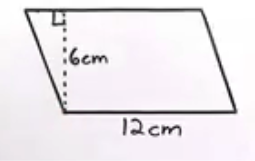Step 2: Calculate the area of the parallelogram. In this case,

12 cm x 6 cm = 72 cm2

Step 3: Instruct students to cut out the parallelogram and cut along the dotted line representing its height. Then, move the triangular piece to the other side of the parallelogram, like this: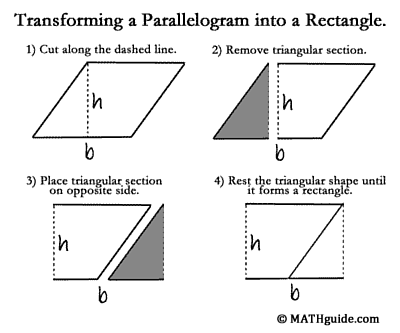Image Source

Step 4: Now we have a rectangle with a base of 12 cm and a height of 6 cm. Again, we calculate the area of the rectangle to be 72 cm2.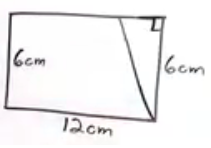We know that the area of the rectangle is exactly the same as the area of the parallelogram because we haven’t added to or taken away from the original parallelogram. We have just rearranged its parts. Therefore, we can be confident that the same formula works for both.

## 4. Demonstrating the Equality of Vertical Angles

When two lines intersect, we get vertical angles. In the image below, angles 1 and 3 are vertical, and angles 2 and 4 are vertical.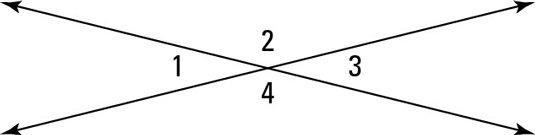Visually, the vertical angles appear to be equal, but we can never assume anything in geometry just by looking. We must prove it.

So how can we do that in this instance?

It turns out that, this one is quite simple. Here’s how to do it:

Step 1: Provide each student a piece of tracing paper. Have the students draw intersecting lines, each of the same length, to create two sets of vertical angles.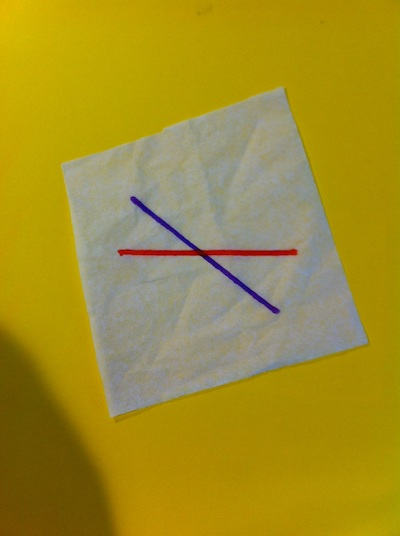Image Source

Step 2: Have the students fold the paper at the point of intersection so that the lines match up. The students will see through the tracing paper that the vertical angles match up, meaning they are equal.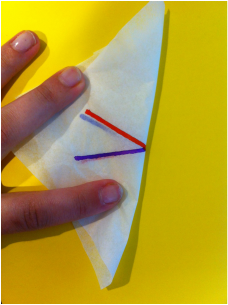Image Source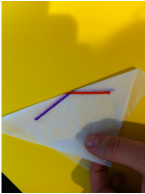Image Source

And, simple as that, we can see that vertical angles are equal!

Tips for success:: In distance learning situations, students may not have access to tracing paper. Other household items that will work include parchment paper, wax paper or the tissue paper used in gift-wrapping.

Once your students have completed this activity, assign them flashcards to help them round out their knowledge of the information.

## 5. Discovering the Pythagorean Theorem

One of the most well-known theorems in geometry, the Pythagorean Theorem states that the square of the hypotenuse of any right triangle  is equal to the sum of the squares of the other two sides, or:

a2+b2=c2Want to make this concept stick?

Again, don’t just tell your students the theorem. Allow them to discover it on their own.  Here’s how:

Step 1: Distribute a variety of right triangles drawn to the following specifications:

• Leg = 3 inches     Leg = 4 inches     Hypotenuse = 5 inches

• Leg = 6 inches     Leg = 8 inches     Hypotenuse = 10 inches

• Leg = 5 cm     Leg = 12 cm     Hypotenuse = 13 cm

• Leg = 8 cm     Leg = 15 cm     Hypotenuse = 17 cm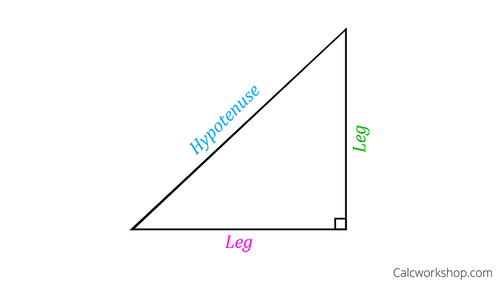Note: Other right triangles made of Pythagorean Triples will also work, but these may need to be drawn on paper larger than standard letter-sized paper or measured in a smaller unit, such as millimeters, in order to fit.

Step 2: Ask the students to measure the three sides of their triangles.

Important! Be sure to indicate the unit (inches, centimeters or millimeters) that students should use to measure each triangle, and be clear that the sides of the triangles are drawn to the nearest unit.

Step 3: Now, instruct the students to take the measurements of the three sides of each triangle to create a balanced equation, using one addition sign, one equals sign, and three exponents of 2.  Using the triangles listed in Step 1, for example, the students will find that they can create these equations:

32+42=52

52+122=132

82+152=172

Each of these equations balances, for example:

32+42=52

9+16=25

25=25

Step 4: Finally, ask students to look for a pattern amongst the different triangles.

Tips for success: Individual students should compare their triangles with their own (if they’ve worked with more than one triangle,) with their group mates' triangles, or with other groups’ triangles to discover that, for every triangle, the pattern is the same: The square of the hypotenuse is equal to the sum of the squares of the other two sides.

This consistent pattern demonstrates the truth of the Pythagorean Theorem.

Again, for distance learning, students may share or upload their work for their classmates to evaluate the pattern.

Students can drive home what they’ve learned in the activity with these flashcards.

## 6. Showing That the Triangle Inequality Theorem is True

The first five activities we’ve discussed cover some of the most basic concepts in geometry. The next two activities explore ideas that come slightly later in most geometry texts or units. We will begin with the Triangle Inequality Theorem

What is the Triangle Inequality Theorem?

This theorem states that the sum of the measures of any two sides of a triangle must be greater than the measure of the third side.

Here’s how students can explore this theorem in a hands-on manner:

Step 1: Give each student or group of students five straws cut to the following lengths:

• 2 inches
• 3 inches
• 4 inches
• 5 inches
• 10 inches

Step 2: Ask the students to construct a triangle by laying the following pieces end to end: the 3 inch piece, the 4 inch piece, and the 5 inch piece. The students will find that they can construct a triangle.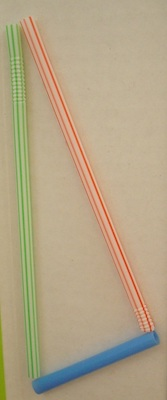Step 3: Now, have the students construct a triangle using the 2-inch piece, the 3-inch piece, and the 5-inch piece. The students will find that this triangle is not possible. See the second attempt at a triangle, below.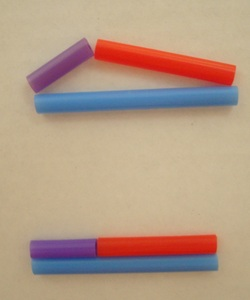Step 4: Then, have the students construct a triangle using the 4-inch piece, the 5-inch piece, and the 10-inch piece. Again, the students will find that this triangle is not possible. It will not close.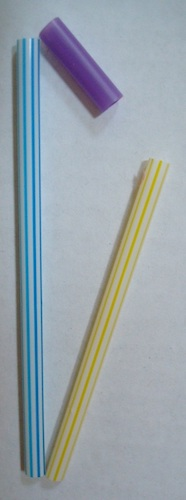Step 5: Finally, have the students write equality and inequality statements for each set of straws.

• The 3-inch, 4-inch piece, and 5-inch set:

3+4>5

3+5>4

4+5>3

• The 2-inch, 3-inch piece, and 5-inch set:

2+3=5

2+5>3

3+5>2

• The 4-inch, 5-inch piece, and 10-inch set:

4+5<10

4+10>5

5+10>4

Step 6: Congratulate your students on having discovered the Triangle Inequality Theorem!

But how did they do it?

Here’s how:

They were only able to construct a triangle using the first set of measurements: 3 inches, 4 inches and 5 inches. The sum of any two of those sides is greater than the remaining side..

However, for the two sets of measurements that cannot create triangles, only two combinations produce such an inequality. In the second case, the combination 2 + 3 produces a sum equal to the third side. In the third case, the combination 4 + 5 produces a sum less than the third side.

Tips for success: Allow students to continue to experiment with this set of straws, or include other lengths of straws, to find additional sets of side lengths that produce triangles according to the Triangle Inequality Theorem.

For distance learning, provide instructions for the lengths to which students must cut their own pieces of straw. In place of straws, other items that can work are string laid out straight, or thin strips of paper cut to about a quarter-inch of thickness and to the correct lengths.

## 7. Proving that the Center of Gravity of a Triangle is Its Centroid

This is a fun activity that combines geometry with a little bit of physics. The centroid  of a triangle is the point at which the three medians of the triangle meet. The centroid is also the center of gravity of a triangle, or the point on which it balances

And we can prove it! Here’s how:

Step 1: On cardboard, have students construct a triangle of their choosing. Then, have them find the centroid by carefully drawing the medians of the triangle. The median of a triangle is a line segment from one vertex to the midpoint opposite it.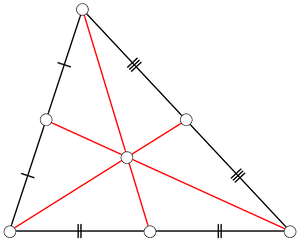Step 2: Once they have found the centroid, ask the students to poke their pencil into the cardboard at the centroid and carefully hold their pencil vertically. The triangle will balance!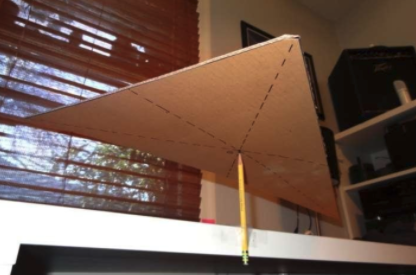Step 3: To demonstrate that the triangle will only balance at the centroid, ask the students to try poking their pencil into other spots on the surface of the triangle. They will find that the triangle does not balance.

Tips for success: If a student’s triangle will not balance, he or she has probably not found the centroid correctly. Have that student go back and re-measure for the midpoints. Then, check that each median accurately connects the midpoint to its opposite vertex in a straight line.

In a remote learning setting, this is a fun activity for students to prepare ahead of time and bring to their virtual session. They can “show off” their balancing triangles.

And, there we have it! Use these seven hands-on, discovery-based activities to help your students explore and discover geometric principles on their own. Whether used in a classroom setting or as part of a virtual classroom, these activities will help them become active learners who understand and recall geometry even better!